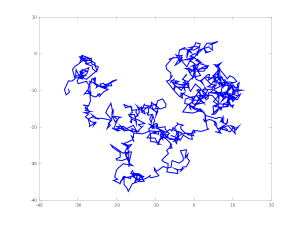It is amusing to realize how a good idea may appear differently in several independent minds during the same period. Moreover, from the scientific point of view, being the first is less important than having an impact.  Regarding Brownian Motion, we find in Wikipedia:

The first person to describe the mathematics behind Brownian motion was Thorvald N. Thiele in a paper on the method of least squares published in 1880. This was followed independently by Louis Bachelier in 1900 in his PhD thesis “The theory of speculation”, in which he presented a stochastic analysis of the stock and option markets. Albert Einstein (in one of his 1905 papers) and Marian Smoluchowski (1906) brought the solution of the problem to the attention of physicists, and presented it as a way to indirectly confirm the existence of atoms and molecules.

Ironically, the microscope observation of the famous (at that time) Scottish Botanist Robert Brown regarding moving particles was anecdotal in his scientific work.

Here are some excerpts from the paper On the motion of small particles suspended in liquids at rest required by the molecular-kinetic theory of heat, by Albert Einstein, published in Annalen der Physik 17 (1905) 549–560. The original paper is in German. An English translation is available in Einstein’s miraculous year: five papers that changed the face of physics.

In this paper it will be shown that, according to the molecular kinetic theory of heat, bodies of a microscopically visible size suspended in liquids must, as a result of thermal molecular motions, perform motions of such magnitude that they can be easily observed with a microscope. It is possible that the motions to be discussed here are identical with so-called Brownian molecular motion; however, the data available to me on the latter are so imprecise that I could not form a judgment on the question.

We shall now turn to a closer examination of the disordered motions that arise from thermal molecular motion and give rise to the diffusion investigated in the last section. Obviously, we must assume that each individual particle executes a motion that is independent of the motions of all the other particles; the motions of the same particle in different time intervals must also be considered as mutually independent processes, so long as we think of these time intervals as chosen not to be too small. We now introduce a time interval τ, which is very small compared with observable time intervals but still large enough that the motions performed by a particle during two consecutive time intervals τ can be considered as mutually independent events. Suppose, now, that a total of n suspended particles is present in a liquid.

Let $f(x,t)$ be the number of particles per unit volume… we get

$$\frac{\partial f}{\partial t}=D\frac{\partial^2 f}{\partial x^2}$$
This is the well-known differential equation for diffusion, and we recognize that D is the diffusion coefficient. We have assumed that the individual particles are all referred to the same coordinate system. However, this is not necessary since the motions of the individual particles are mutually independent. … The problem, which coincides with the problem of diffusion outwards from a point (neglecting the interaction between the diffusing particles), is now completely determined mathematically; its solution is
$$f(x,t)=\frac{n}{\sqrt{4\pi D}}\frac{e^{-\frac{x^2}{4Dt}}}{\sqrt{t}}.$$
The probability distribution of the resulting displacements during an arbitrary time t is thus the same as the distribution of random errors, which was to be expected. What is important, however, is how the constant in the exponent is related to the diffusion coefficient.

Further, we found in section 4 that the mean value of the displacements of the particles in the X-direction at time t equals $\lambda_x=\sqrt{2Dt}$.

We will now calculate how large $\lambda_x$ is for one second if $N$ is taken to be $6 \times 10^{23}$ in accordance with the results of the kinetic theory of gases; water at 17° C ($k = 1.35\times 10^{-2}$) is chosen as the liquid, and the diameter of the particles is $0.001$ mm. We get $\lambda_x = 8 \times 10^{-5}$ cm $= 0.8$ micron. Therefore, the mean displacement in one minute would be about 6 microns.

Note: regarding Brownian Motion and stochastic modeling in finance, you may read the book entitled The (mis)behaviour of markets: a fractal view of risk, ruin and reward by Mandelbrot and Hudson, in particular Chapter XII entitled Ten heresies of finance.

Further reading: Sur la théorie du mouvement brownien, by Paul Langevin, 1908. Excerpt: «… J’ai pu constater tout d’abord qu’une application correcte de la méthode de M. Smoluchowski conduit à retrouver la formule de M. Einstein exactement et, de plus, qu’il est facile de donner, par une méthode toute différente, une démonstration infiniment plus simple. … »

Last Updated on

This site uses Akismet to reduce spam. Learn how your comment data is processed.

Syntax · Style · .